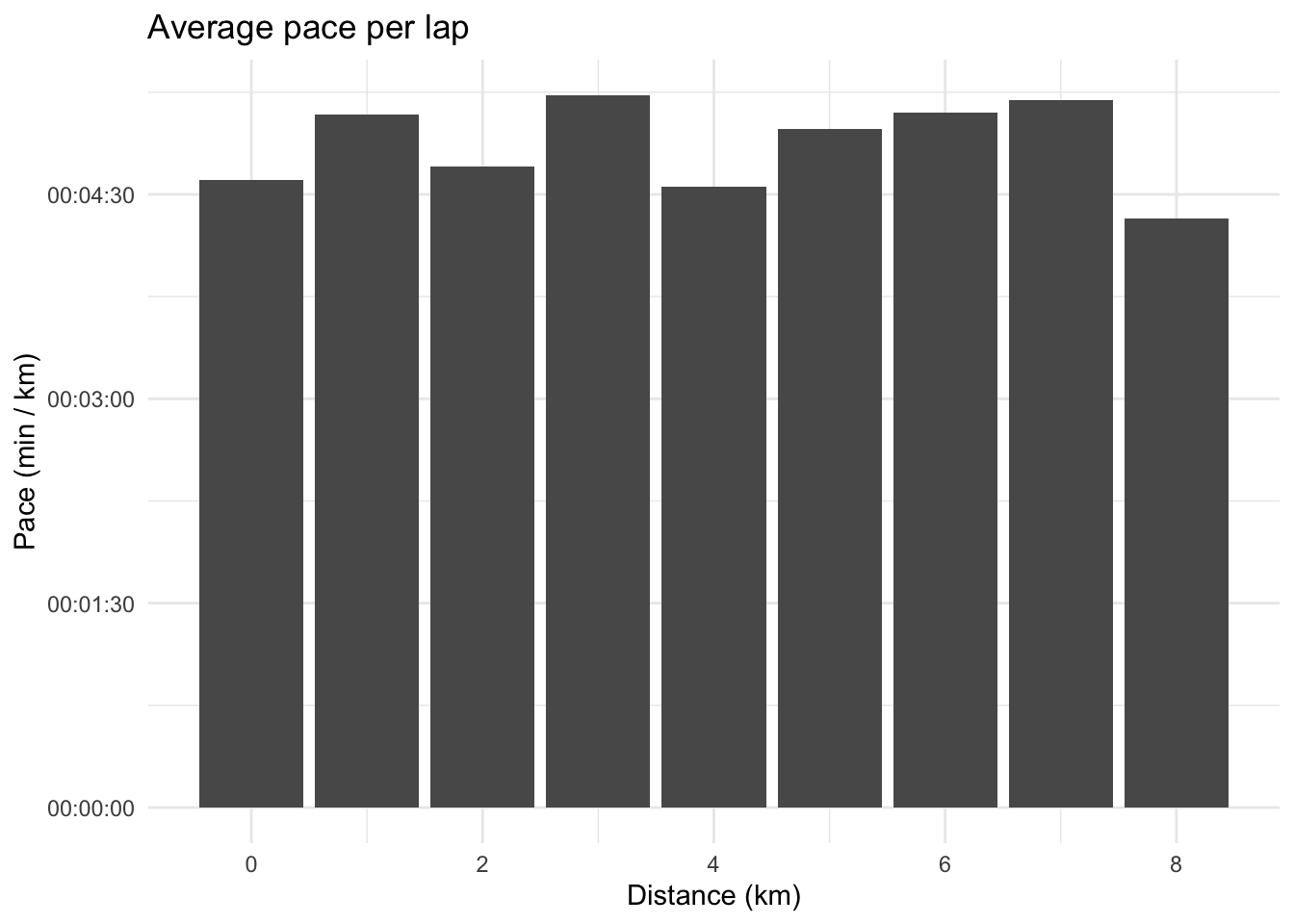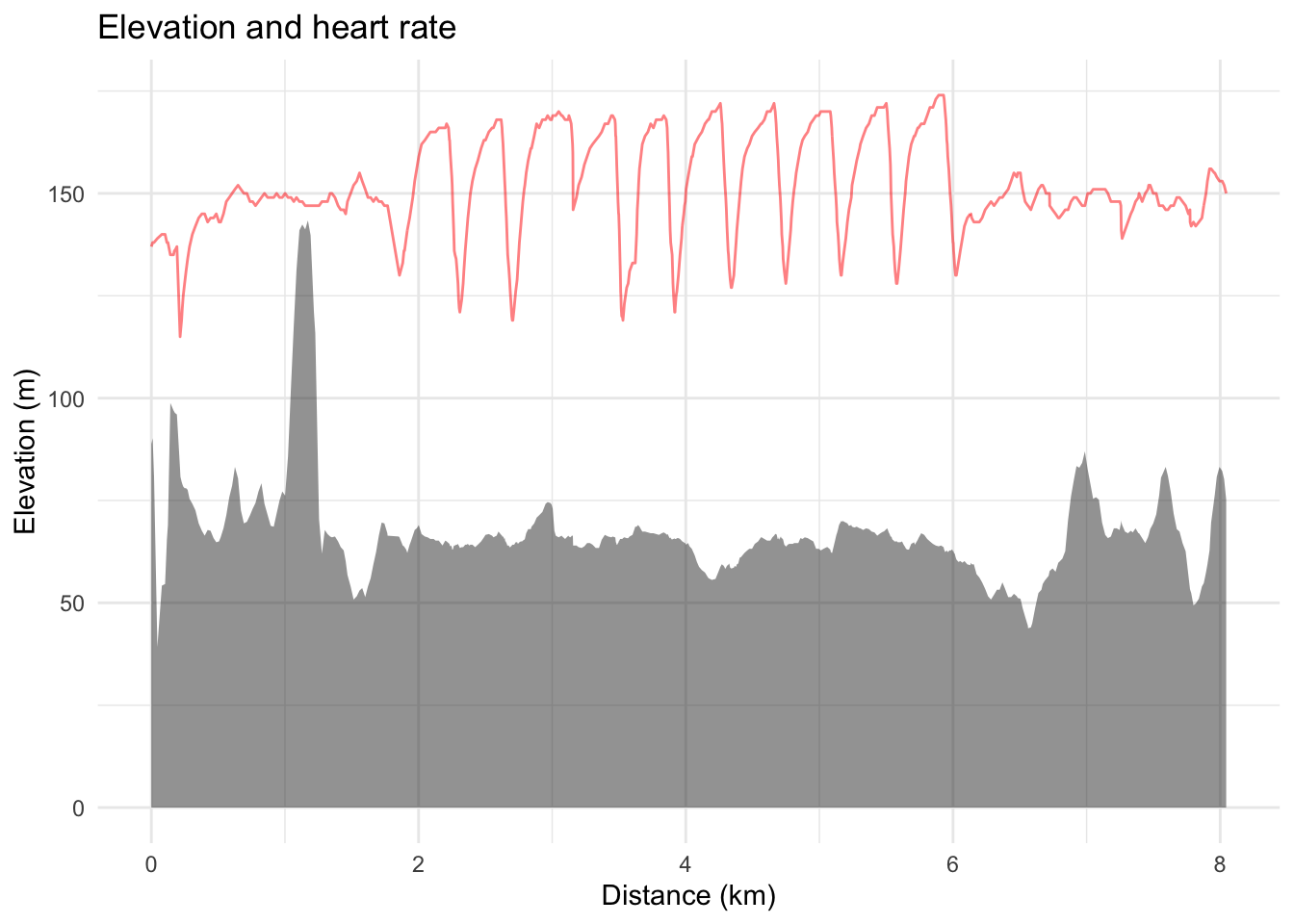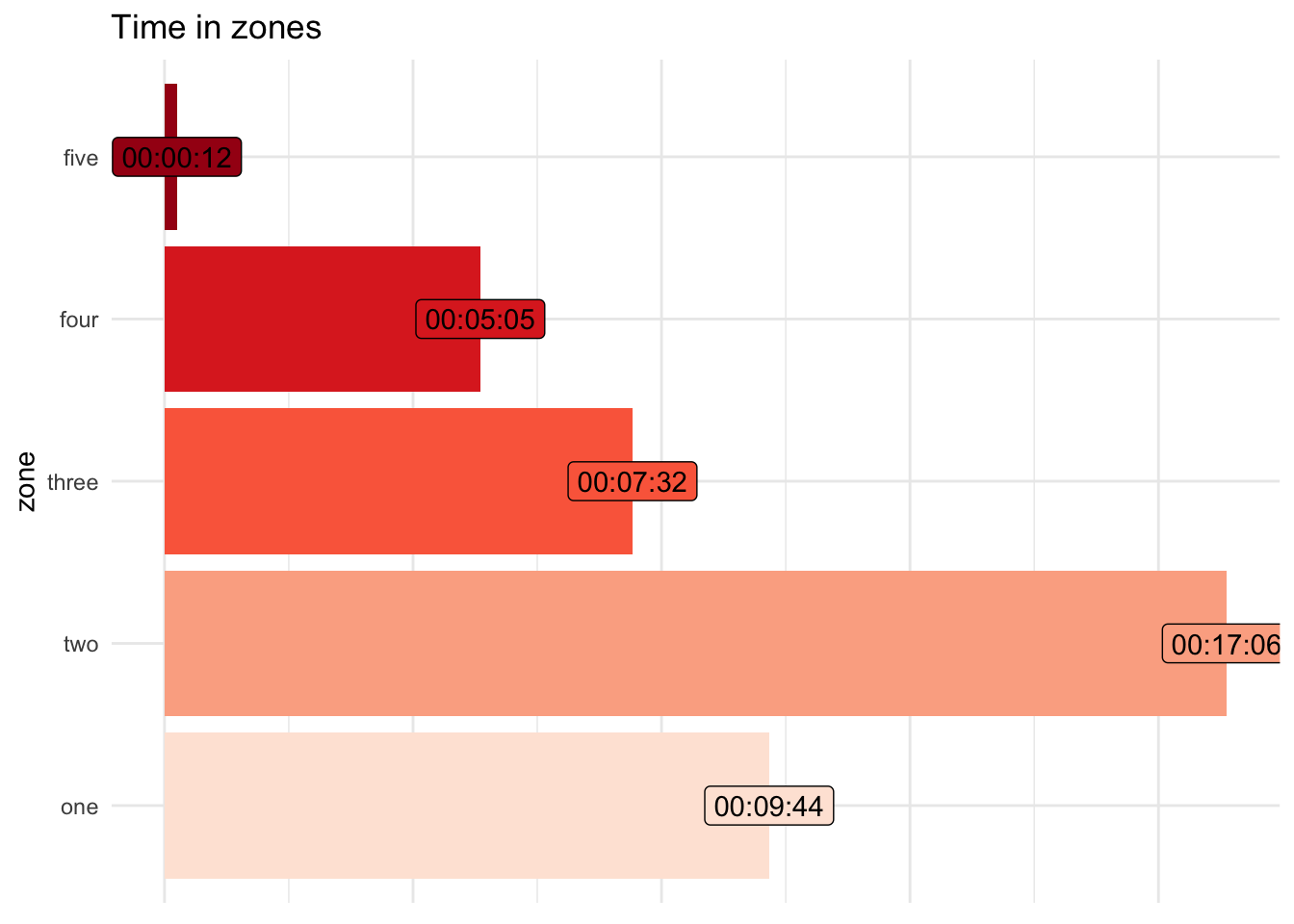# Analysing .fit files in R

R
Author

Jonny Law

Published

November 4, 2019

library(tidyr)
Warning: package 'tidyr' was built under R version 4.1.2
library(dplyr)
Warning: package 'dplyr' was built under R version 4.1.2

Attaching package: 'dplyr'
The following objects are masked from 'package:stats':

filter, lag
The following objects are masked from 'package:base':

intersect, setdiff, setequal, union
library(readr)
Warning: package 'readr' was built under R version 4.1.2
library(ggplot2)
library(reticulate)
Warning: package 'reticulate' was built under R version 4.1.2
library(leaflet)
Warning: package 'leaflet' was built under R version 4.1.2
theme_set(theme_minimal())

Garmin running watches output a file type called .fit, the developer SDK can be downloaded from the ANT website. There is also Python library named fitparse which has been written to parse .fit files. This blog post will show you how to use reticulate to parse a .fit file.

First create a Python virtual environment, this is commonly used to store a projects’ package collection together to enable more straightforward reproducibility. A virtual environment also contains its own Python and the python package manager pip for installing and managing packages. reticulate has a function to create a virtual environment:

virtualenv_create("r-reticulate")
virtualenv: r-reticulate
use_virtualenv("r-reticulate")

## Parsing

The virtual environment can be used to install the Python package fitparse

py_install("fitparse")

The library can be imported as an R object.

fitparse <- reticulate::import("fitparse")

Then methods and classes defined in the fitparse Python libary can be accessed using the $ notation. Typing $ after fitparse (and hitting the TAB key) in the RStudio IDE gives a list of top-level methods and classes defined in the fitparse library.

fit_file <- here::here("posts/2019-11-04-parsing-strava/1001800515.fit")
ff <- fitparse$FitFile(fit_file) We can use the get_messages method on the FitFile. This returns a generator, this is a special type of lazy list in Python. generator <- ff$get_messages("record")

iterate is a function provided by the reticulate library which can be used to traverse a Python generator:

activity <- reticulate::iterate(ff$get_messages("record"), function(x) x$get_values())

This evaluates the generator and applies the function get_values to retrieve the details associated with this activity. A list object is returned by R, the first element looks like this:

activity[]
$timestamp 2017-03-16 17:22:08$position_lat
 655852851

$position_long  -19374352$distance
 3.47

$enhanced_altitude  88.8$altitude
 88.8

$enhanced_speed  3.471$speed
 3.471

$vertical_oscillation  120$stance_time_percent
 34.25

$stance_time  259$heart_rate
 137

$cadence  79$activity_type
 "running"

$fractional_cadence  0 We want to transform this list of lists into a dataframe. The most straightforward way is to extract the elements of interest using the map function from purrr: (activity_tibble <- activity %>% purrr::map_dfr(function(x) tibble( timestamp = readr::parse_datetime(as.character(x$timestamp)),
latitude = x$position_lat, longitude = x$position_long,
elevation = x$enhanced_altitude, heart_rate = x$heart_rate,
cadence = x$cadence ))) # A tibble: 611 × 6 timestamp latitude longitude elevation heart_rate cadence <dttm> <int> <int> <dbl> <int> <int> 1 2017-03-16 17:22:08 655852851 -19374352 88.8 137 79 2 2017-03-16 17:22:09 655853310 -19375987 90.2 138 79 3 2017-03-16 17:22:10 655854123 -19374810 81.2 138 80 4 2017-03-16 17:22:15 655856545 -19372657 39.2 139 78 5 2017-03-16 17:22:21 655859405 -19368861 54.2 140 79 6 2017-03-16 17:22:26 655860948 -19365257 54.6 140 80 7 2017-03-16 17:22:32 655860904 -19362723 65.4 138 79 8 2017-03-16 17:22:33 655861059 -19361314 69 138 79 9 2017-03-16 17:22:37 655860087 -19358357 98.8 135 79 10 2017-03-16 17:22:44 655860947 -19354267 97 135 80 # … with 601 more rows Notice that the latitude and longitude don’t look correct, it turns out they are in semicircles and can be converted to a recognisable coordinate system using the following function. semicircle_to_degrees <- function(semicircle) semicircle * (180 / 2**31) Applying the function to the latitude and longitude models. activity_tibble <- activity_tibble %>% mutate_at(vars(latitude, longitude), semicircle_to_degrees) This is a very basic summary of the activity. We can derive the distance per timestep using the longitude, latitude and timestamp fields. (activity_tibble <- activity_tibble %>% mutate( time_diff_to_prev = as.numeric(difftime(timestamp, lag(timestamp, default = .$timestamp))),
cumtime = cumsum(time_diff_to_prev),
dist_to_prev = c(0, sp::spDists(
x = as.matrix(.[, c("longitude", "latitude")]),
longlat = TRUE,
segments = TRUE
)),
elevation_to_prev = elevation - lag(elevation),
distance = cumsum(dist_to_prev)
)) 
# A tibble: 611 × 11
timestamp           latitude longitude elevation heart_rate cadence
<dttm>                 <dbl>     <dbl>     <dbl>      <int>   <int>
1 2017-03-16 17:22:08     55.0     -1.62      88.8        137      79
2 2017-03-16 17:22:09     55.0     -1.62      90.2        138      79
3 2017-03-16 17:22:10     55.0     -1.62      81.2        138      80
4 2017-03-16 17:22:15     55.0     -1.62      39.2        139      78
5 2017-03-16 17:22:21     55.0     -1.62      54.2        140      79
6 2017-03-16 17:22:26     55.0     -1.62      54.6        140      80
7 2017-03-16 17:22:32     55.0     -1.62      65.4        138      79
8 2017-03-16 17:22:33     55.0     -1.62      69          138      79
9 2017-03-16 17:22:37     55.0     -1.62      98.8        135      79
10 2017-03-16 17:22:44     55.0     -1.62      97          135      80
# … with 601 more rows, and 5 more variables: time_diff_to_prev <dbl>,
#   cumtime <dbl>, dist_to_prev <dbl>, elevation_to_prev <dbl>, distance <dbl>

## Summarising

We can calculate a high level summary of the activity similar to what you would find on Garmin connect.

activity_tibble %>%
summarise(
total_distance = sum(dist_to_prev),
elapsed_time = max(timestamp) - min(timestamp),
moving_time = sum(time_diff_to_prev) - sum(
ifelse(dist_to_prev == 0, time_diff_to_prev, 0)
),
elevation_gain = sum(if_else(elevation_to_prev > 0, elevation_to_prev, 0), na.rm = TRUE),
average_heart_rate = round(mean(heart_rate), 0)
) %>%
mutate(average_pace = hms::as_hms(as.numeric(moving_time) / total_distance),
moving_time = lubridate::seconds_to_period(moving_time)) %>%
knitr::kable()
total_distance elapsed_time moving_time elevation_gain average_heart_rate average_pace
8.044634 39.65 mins 39M 39S 380 150 00:04:55.72509

We can recreate plots commonly found on activity websites such as training peaks, Strava and Garmin Connect, for instance average speed for each 1km:

activity_tibble %>%
mutate(lap = distance %/% 1) %>%
group_by(lap) %>%
summarise(lap_distance = sum(dist_to_prev),
lap_time = sum(time_diff_to_prev),
pace = hms::as_hms(lap_time / lap_distance)) %>%
ggplot(aes(x = lap, y = pace)) +
geom_col() +
xlab("Distance (km)") +
ylab("Pace (min / km)") +
labs(title = "Average pace per lap")activity_tibble %>%
mutate(distance = cumsum(dist_to_prev)) %>%
ggplot(aes(x = distance, y = elevation)) +
geom_area(alpha = 0.5) +
geom_line(aes(x = distance, y = heart_rate), colour = "#ff0000", alpha = 0.5) +
xlab("Distance (km)") +
ylab("Elevation (m)") +
labs(title = "Elevation and heart rate")## Analysing heart rate data

We can determine how hard the activity was for the athlete using heart rate data. Heart rate is an individual metric and differs between athletes running the same pace. To that end, we must compute the heart rate relative to the maximum heart rate or using heart rate reserve (taking into account both the maximum and resting heart rate). Using the max heart rate and resting heart rate, training zones can be determined. These zones are broad and for the convenience of the athlete (and coach) when performing workouts at a given intensity. This intensity should vary depending on the purpose of the workout (recovery, threshold, VO2 max intervals etc.).

Suggested heart rate zones according to Pete Pfitzinger are:

1. Active recovery: less than 76% MHR
2. General Aerobic: 70%-81% MHR
3. Tempo (Marathon pace): 81%-88% MHR
4. Lactate Threshold: 82%-92% MHR
5. Anaerobic: 95%+

For my maximum heart rate of 189, the zones can be written as.

zones <- c(
"one" = 0.76 * 189,
"two" = 0.81 * 189,
"three" = 0.88 * 189,
"four" = 0.92 * 189,
"five" = 189)
knitr::kable(tibble::rownames_to_column(data.frame(heart_rate = round(zones, 0)), var = "zone"))
zone heart_rate
one 144
two 153
three 166
four 174
five 189

Then the time in zones can be plotted for the given activity.

time_in_zones <- activity_tibble %>%
mutate(
zone = dplyr::case_when(
heart_rate <= zones ~ names(zones),
heart_rate <= zones ~ names(zones),
heart_rate <= zones ~ names(zones),
heart_rate <= zones ~ names(zones),
heart_rate <= zones ~ names(zones),
)
) %>%
mutate(zone = factor(zone, levels = c("one", "two", "three", "four", "five"))) %>%
group_by(zone) %>%
summarise(time_seconds = sum(time_diff_to_prev))

time_in_zones %>%
ggplot(aes(x = zone, y = time_seconds, fill = zone)) +
geom_col() +
scale_fill_brewer(type = "seq",
direction = 1,
palette = "Reds") +
scale_x_discrete(drop = FALSE) +
theme(legend.position = "none") +
theme_minimal() +
coord_flip() +
scale_y_time() +
geom_label(aes(label = hms::as_hms(time_seconds))) +
theme(
axis.title.x = element_blank(),
legend.position = "none",
axis.ticks.x = element_blank(),
axis.text.x = element_blank()
) +
labs(title = "Time in zones")## Training impulse

TRIMP can be used (TRaining IMPulse) to calculate a one-number summary of the activity difficulty, more information on TRIMP can be found here.

The most straightforward way to calculate TRIMP is calculating the total time in each zone by multiplying the zone number by the total minutes in the corresponding zone.

time_in_zones %>%
summarise(trimp_zone = sum(as.numeric(zone) * time_seconds / 60)) %>%
knitr::kable()
trimp_zone
87.86667

This number is straightforward to calculate however it lacks nuance. For instance it remains the same if the athlete is at either the upper or lower end of the heart rate range for a given zone. To account for this TRIMP exp can be calculated:

$\textrm{TRIMP}^{\textrm{exp}} = \sum_{i=1}^T \textrm{D}_i \cdot \textrm{HRr} \cdot 0.64e^y$

Where, $$\textrm{D}_i$$ is the duration of a single measurement (typically one to five seconds on a Garmin watch), HRr is the heart rate reserve (maximum heart rate - resting heart rate), $$y$$ is the percentage of heart rate reserve multiplied by 1.92 for men and 1.67 for women.

trimp_exp <- function(heartrate, time_seconds, max_hr, resting_hr, sex = "Male") {
heart_rate_reserve <- max_hr - resting_hr
hrr <- heartrate / heart_rate_reserve
constant <- if_else(sex == "Male", 1.92, 1.67)
sum((time_seconds / 60) * hrr * 0.64 * exp(constant * hrr))
}
activity_tibble %>%
summarise(trimp_exp = trimp_exp(heart_rate, time_diff_to_prev, 189, 42)) %>%
knitr::kable()
trimp_exp
187.6727

These summaries can be used to calculate the overall training workload for an athlete to assist with planning and reviewing training plans. This is typically used in addition to training time and distance covered.

## Citation

BibTeX citation:
@online{law2019,
author = {Jonny Law},
title = {Analysing .fit Files in {R}},
date = {2019-11-04},
langid = {en}
}